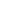# Make ten when one addend is 8.

EngageNY 60 min(s)
In this lesson, students use linking cubes to add 8 and another single-digit number by decomposing the second addend to make ten with the 8. For example, they solve the problem "Peter has 8 books, and Willie has 5. How many books do they have altogether?" by making two groups (of 8 and 5), moving two cubes over from the 5 to make a ten, and then adding 10 + 3 to find the solution.